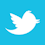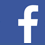Understand what is happening to the energy moving around in a circuit board.

I want to challenge your understanding of electricity. My intentions are honorable. Technology is asking us to move more and more data, which in turn requires the use of more and more bandwidth. The tools we use have evolved from circuit theory, where we were very comfortable using lumped parameter models. Today, the interconnecting structures on logic circuit boards are just as important as the components. How a board is laid out controls delays, radiation and bit rates. I feel the meaning of voltage when it involves fast logic needs to be given a closer look. That is the subject of this article.

The measurements we make in circuits involve voltage differences. Logic is usually the presence or absence of voltage on a transmission line. We also measure radiation levels using antennas, which convert fields to voltage. We are totally immersed in describing electrical activity in terms of voltage. As we add bandwidth, it is important to review what a voltage measurement might imply. It is our window into electrical activity. I think I’m going to surprise you.

I often tell engineers that nature does not read labels. I might add that nature does not read our textbooks or equations. It turns out engineers need voltage, but nature pays no heed to this concept. For many practical reasons, voltage is a tool that’s here to stay. There is no way to make progress without understanding what a voltage difference can imply when wave action is involved. We can predict what the voltage patterns might be, but unfortunately we cannot connect to most of the conducting surfaces that are involved. On a practical basis, we can observe only one point-pair at a time. On top of all this, things happen at the speed of light. If you really want to be impressed, there are relativistic effects to be considered.

##### Voltage – The Definition and the Problem

To define voltage, it is necessary to introduce the idea of field. We all live in a gravity field that attracts our body mass. Fields store energy. As an example of energy storage, the moon/earth system stores the energy that give us our tidal action. Fields inside an atom form the nucleus. The energy in the nucleus of the atom is enormous.

A collection of surplus electrons on a small mass creates an electric field. Voltage is defined as the work required to move a unit test charge in this field.

Voltage is not a point concept. There are only voltage differences. Voltage differences can exist between points in space, as well as between conductors.

On a dry day, the friction of shoe leather on a rug allows the body to pick up a charge that causes an arc from a key to a door lock. This excess charge is measured as a voltage between the body and the door. The rubbing shoe leather does the work that moves the electric charge to the body. When the voltage gradient is high enough, there is arcing in air. On a grand scale, rain pulls charge from the air to earth. When the voltage gradient is high enough, lightning results.

“Understanding energy is key to designing boards that function without radiation at increasing clock rates.”

In a typical logic circuit, a logic switch connects a voltage source to a short transmission line. This can be a trace over a conducting plane. The presence of a voltage difference means there is an electric field between the two conductors. This field stores energy. When current starts to flow, it creates a coupled magnetic field that uses this same space. A full explanation of what happens is complex and requires several chapters in a book. After all wave activity has stopped, the capacitance of this conductor geometry is fully charged, and the voltage along the transmission line is constant. This means the work required to move a test charge along any path between the two conductors is a constant. You will notice I didn’t discuss the time it takes to make this measurement. One thing for sure is a measurement of work cannot be made in zero time. In the time it takes to make a measurement, wave action may have changed the answer. This is the problem when picoseconds are important.

The difficulty is voltage is not a point concept. Any measure of voltage involves a loop area. This in turn means a voltage measurement depends on lead dress, as well as lead position. This also means many sources can contribute to one observation. The physics of electricity involves point concepts, and this avoids the issue of doing work. The parameters used in physics cannot be observed directly, and this poses a fundamental problem in engineering. Voltage has served us well, but progress requires we get closer to the fundamentals of electricity. We still require voltage, but at the same time we must understand what is happening to the energy moving around in our circuits.

##### Moving Energy

Nature has one main objective and that is to reduce potential energy. It is why a rock falls, sound propagates, ocean waves travel, and why a voltage source will charge a capacitance. Energy is never lost. It simply takes on new forms. On a logic board, a logic level is a voltage on the capacitance of a trace over a ground plane. This voltage implies energy storage. This energy cannot be returned to the power source. To return the voltage to zero, stored energy must be converted to heat, radiated or moved out of the way. Moving energy into position or dissipating energy takes time, and this is the problem encountered in fast logic.

A circuit board trace over a ground plane is an opportunity for nature to reduce potential energy. This reduction in potential energy usually implies spreading the energy out over a larger volume of space. Recognizing this one fact is important in circuit board design. We are interested in manipulating voltages, and nature is only interested in reducing potential energy. What we need to recognize is that when there are voltage differences, there is energy present. At any one point in space, energy can be stationary, in transition, in motion or perhaps all three at once. We are asked to deduce what is happening over an entire transmission line from a limited set of observations. Fortunately, circuit board operations are very repetitive. Once we see the patterns, the work has been done.

##### Voltage Observations

To illustrate the problem of interpreting voltage, consider a transmission line just after a switch connects a voltage source to the line. Assume a finite rise time. Behind the wave front, the voltage is constant. In the space between the two conductors there is both energy flow and energy storage. The stored part of the energy is in the capacitance of the line. The energy that is moving involves both the static electricity and magnetic fields. The voltage alone does not tell this story. The voltage in transition at the leading edge is where magnetic field energy is converted to static electric field energy. After a reflection at an open gate, the voltage doubles. What is happening is energy is still moving forward on the line. Energy is not reflected at the open end of the line. The reflected wave front continues to convert arriving field energy into electric field energy. This is why the voltage doubles on the return wave. This is an example of where the energy flow and wave action are moving in opposite directions.

What I have described is a typical problem in moving energy on a logic circuit board. We know what is happening, but it is not obvious by simply observing voltage. A static voltage difference can represent either energy flow or storage or both. Wave direction and energy flow direction may be opposites.

##### Fast Circuit Boards and Energy Management

This article is intended as an introduction to what takes place on a logic circuit board. Energy is stored and moved in spaces and directed by conductor geometry. This understanding is key to designing boards that will function without radiation at increasing clock rates. Logic by its very nature implies treating transient behavior while carrier systems use sine waves, and this implies a steady-state behavior. Transmission line theory is usually taught in terms of sine waves, where measurement is a well understood discipline. Logic implies transient behavior, and the motion of energy is not that obvious.

John Wiley has published my latest book titled Fast Circuit Boards and Energy Management. The principles outlined in the book help designers meet performance requirements. Basic physics is presented along with simple explanations. This subject of voltage is carefully treated.

Ralph Morrison has 50 years of experience in electronics engineering and is author of eight books, including Solving Interference Problems in Electronics, Grounding and Shielding Techniques in Instrumentation, and The Fields of Electronics: Understanding Electronics Using Basic Physics; This email address is being protected from spambots. You need JavaScript enabled to view it..

Register now for PCB West, the largest trade show for the printed circuit and electronics industry in the Silicon Valley! Coming Sept. 11-13 to the Santa Clara Convention Center.